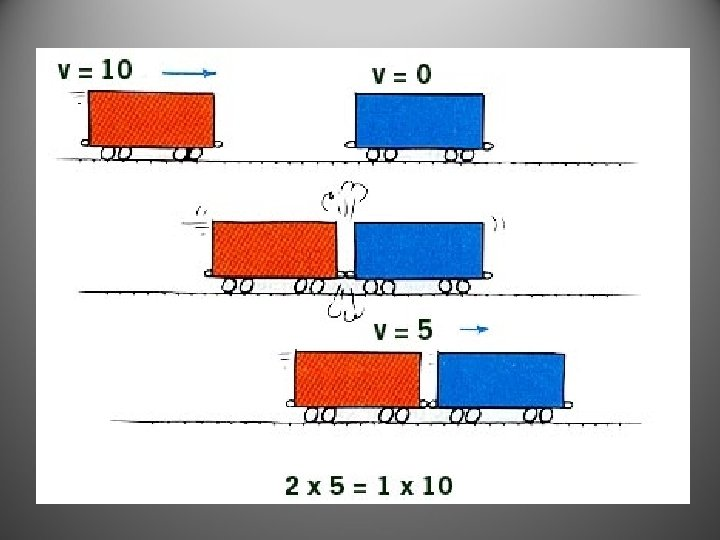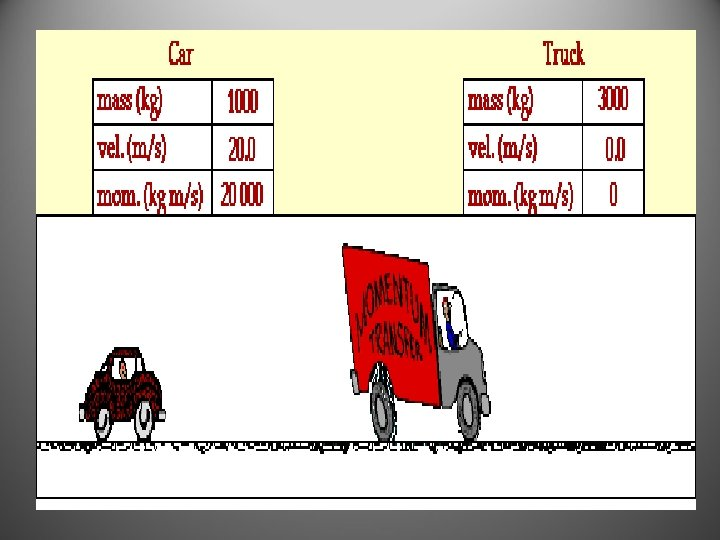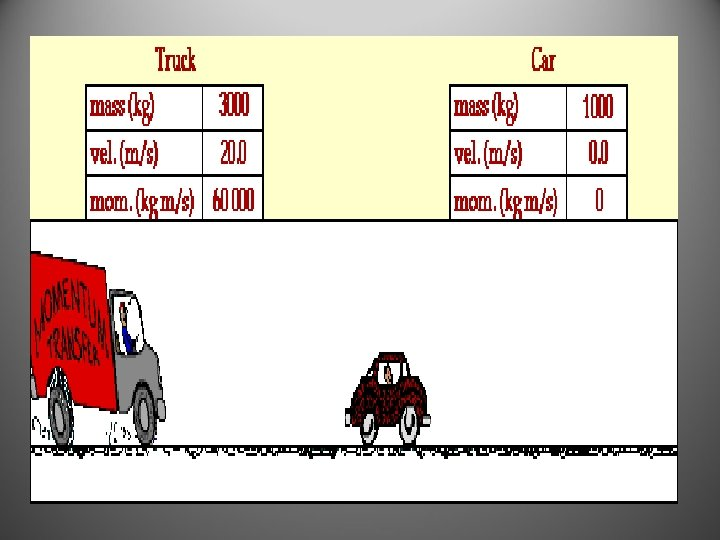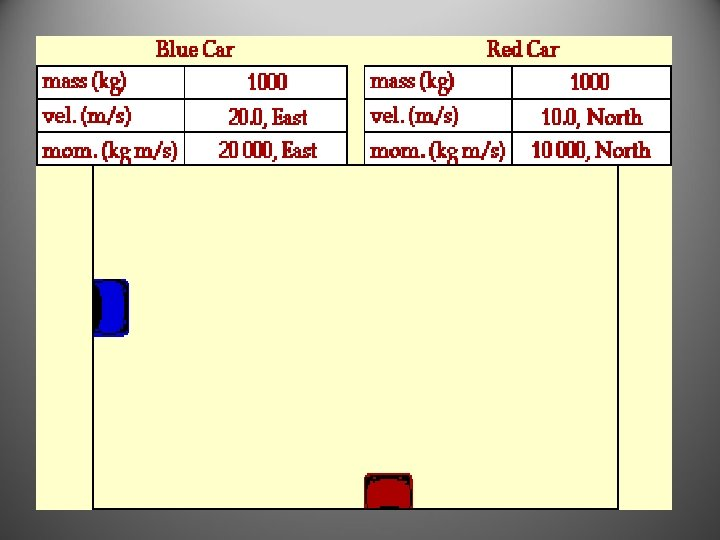# Forces Force and Net Force Force is a

• Slides: 65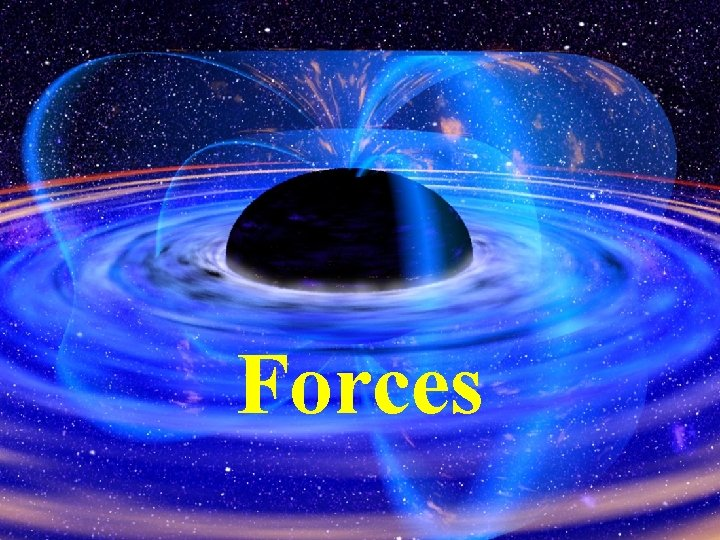Forces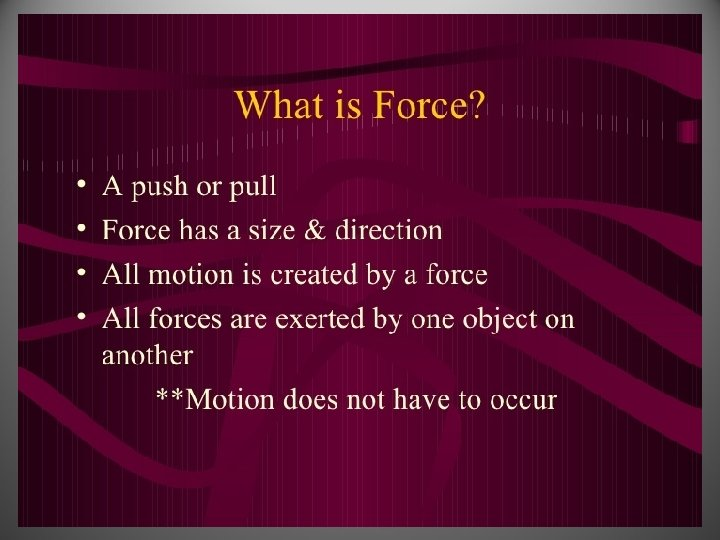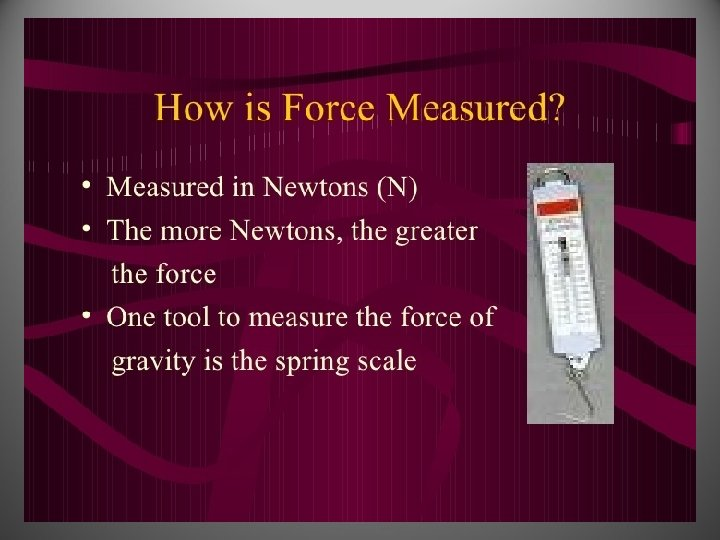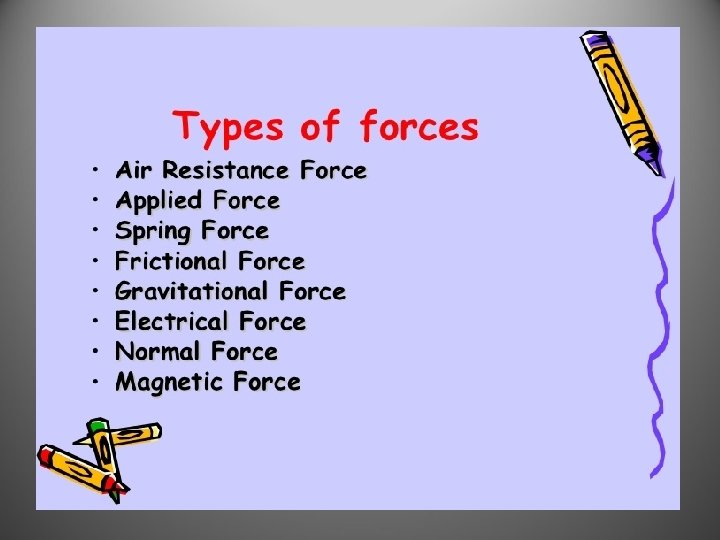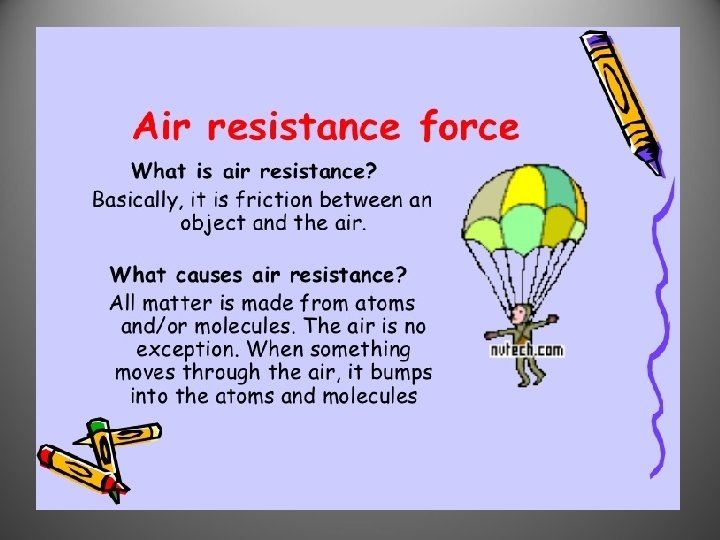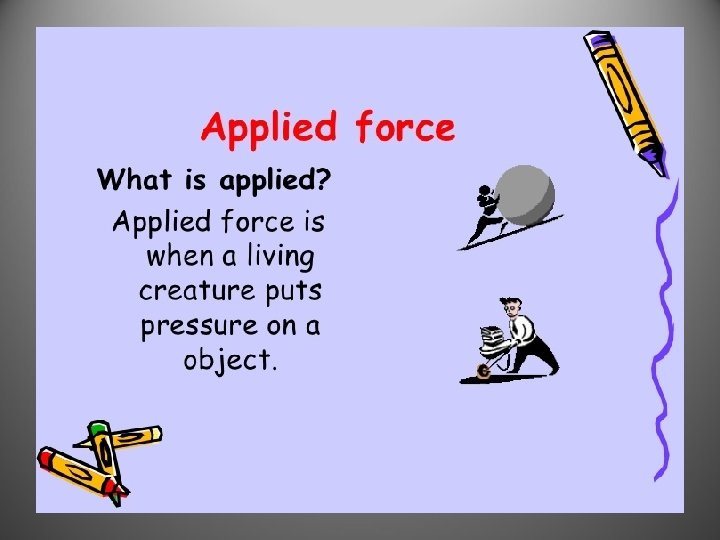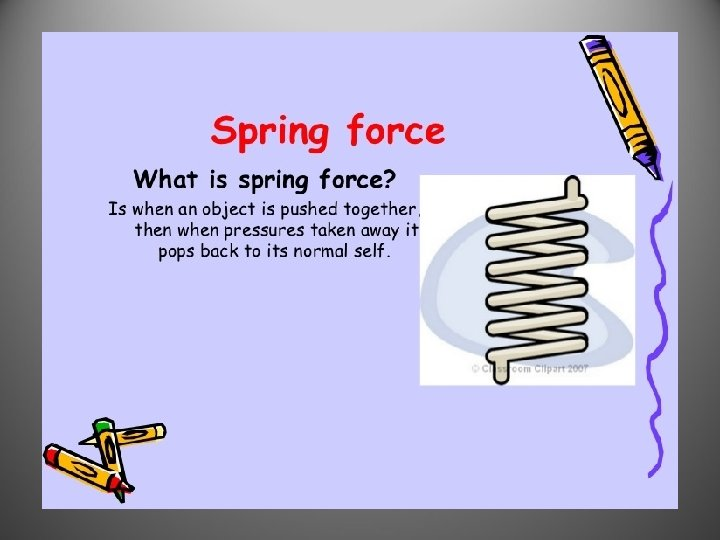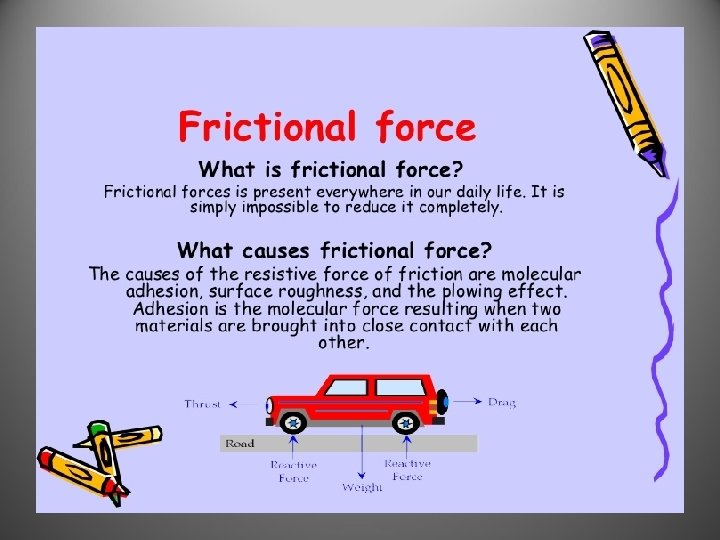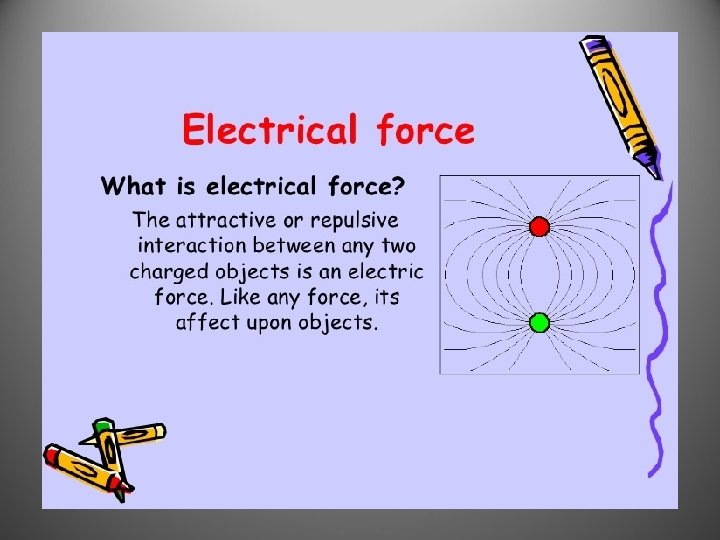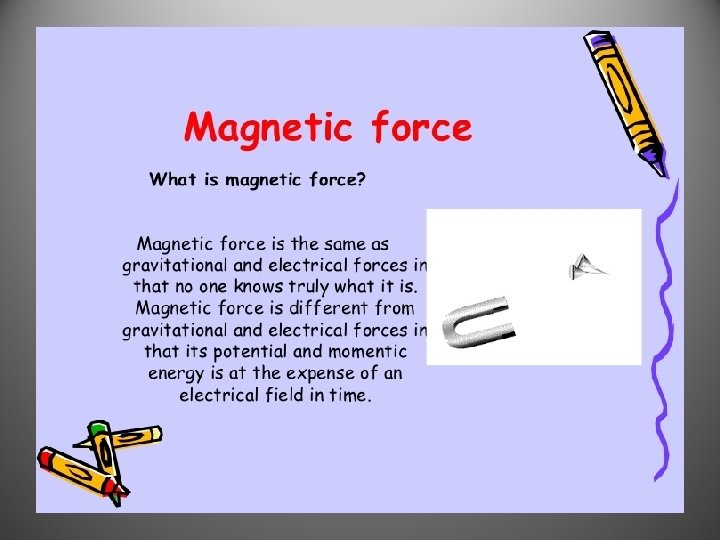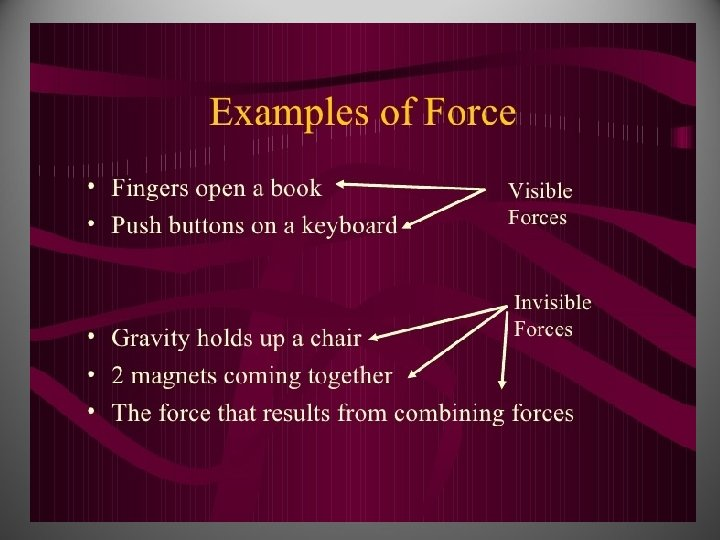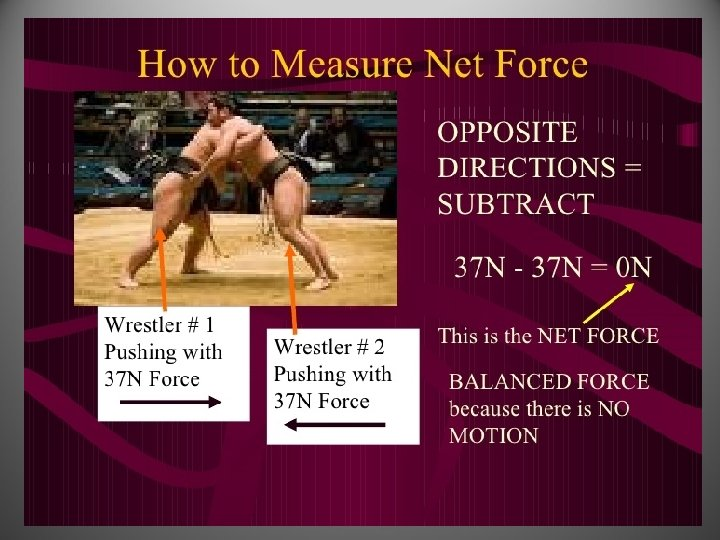Force and Net Force • Force is a push or a pull on an object. • Net force is the total force on an object.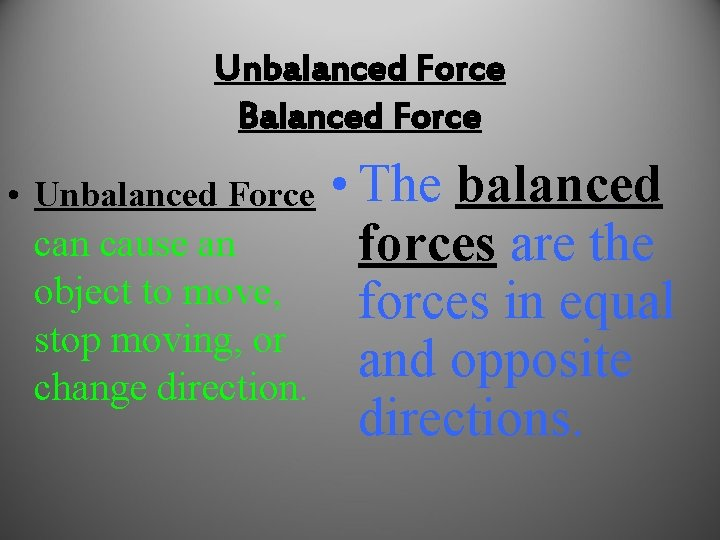Unbalanced Force Balanced Force • Unbalanced Force can cause an object to move, stop moving, or change direction. • The balanced forces are the forces in equal and opposite directions.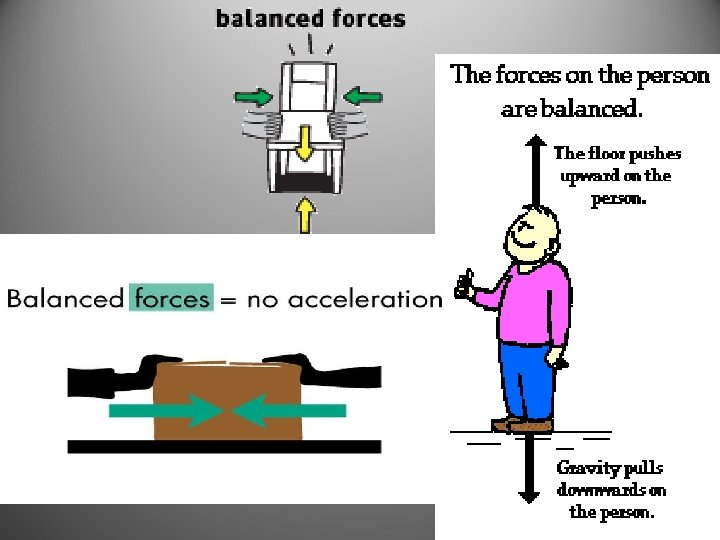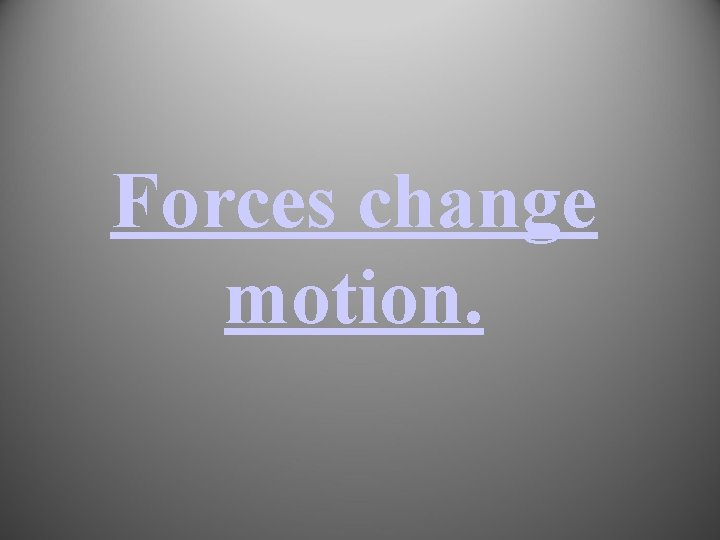Forces change motion.Newton’s First Law Inertia – An object at rest Greater mass will stay at rest means unless acted upon by an outside force. Greater inertia – Inertia is every objects resistance to change its motion.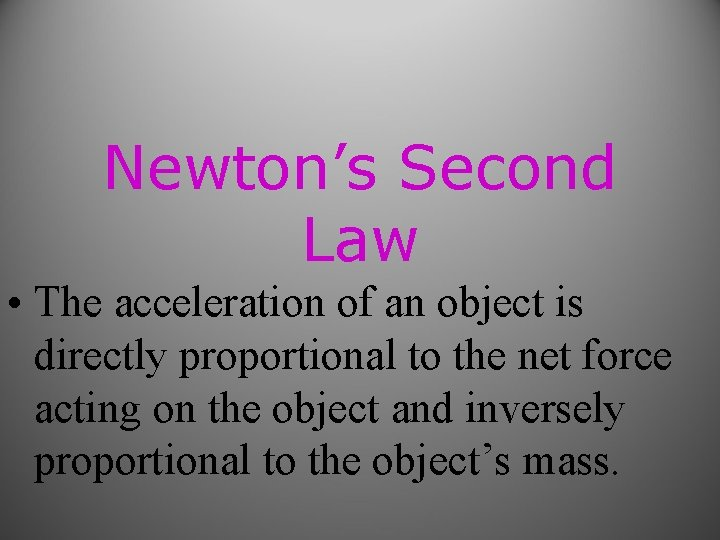Newton’s Second Law • The acceleration of an object is directly proportional to the net force acting on the object and inversely proportional to the object’s mass.Force=mass x acceleration F=m x a • Or • a = Force/ mass • a = F/mEquation for Newton It’s the same as the force equation! • Newton=m x a.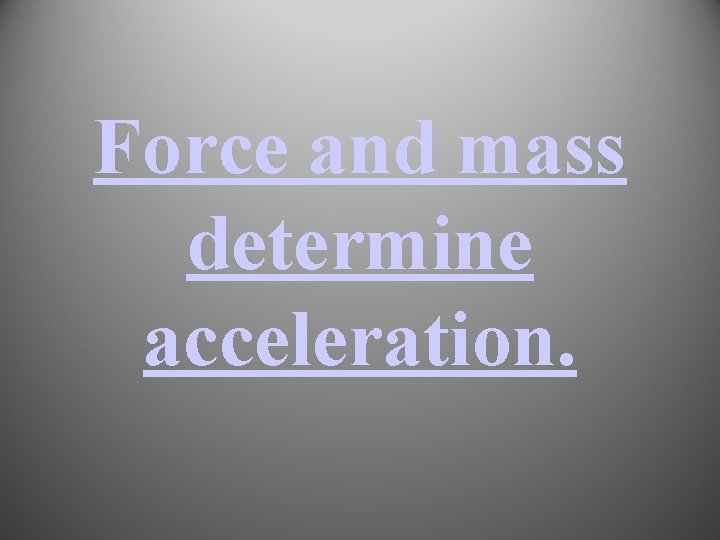Force and mass determine acceleration.Sometimes we might calculate mass using Force and Acceleration • Mass=Force/Acceleration • m=F/aHow much Force was required to make a 2000 kg car accelerate at 5 m/s? 1. 400 N 2. 0. 001 N 3. 10, 000 N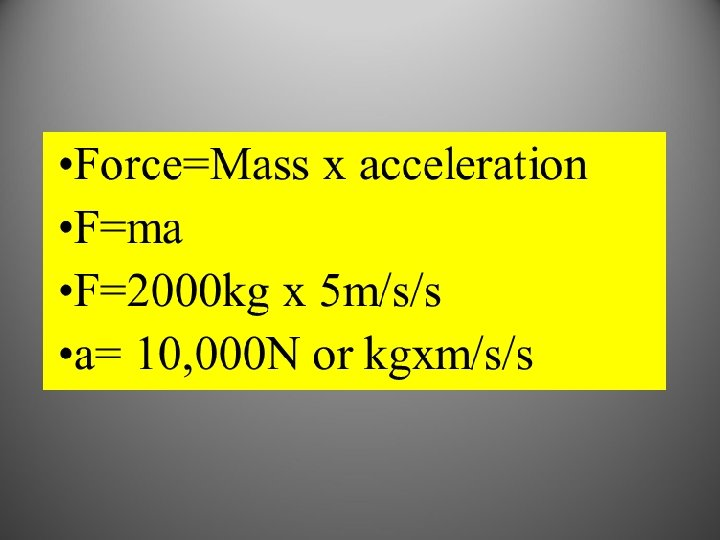If you drop a 15 kg bowling ball with a Force of 147 N, what is it’s acceleration? 1. 9. 8 m/s/s 2. 0. 1 m/s/s 3. 2, 205 m/s/s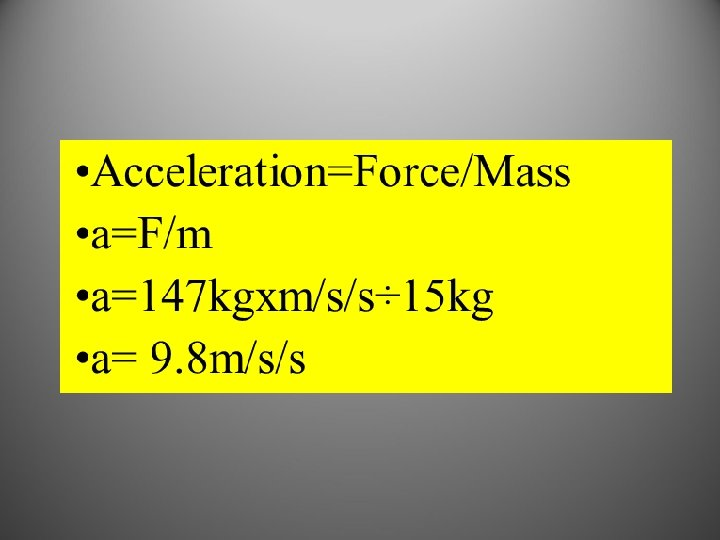A person throws a baseball at an acceleration of 25 m/s/s with 12. 5 N of Force. What’s the Mass of the ball? 1. 312 kg 2. . 5 kg 3. 2 kg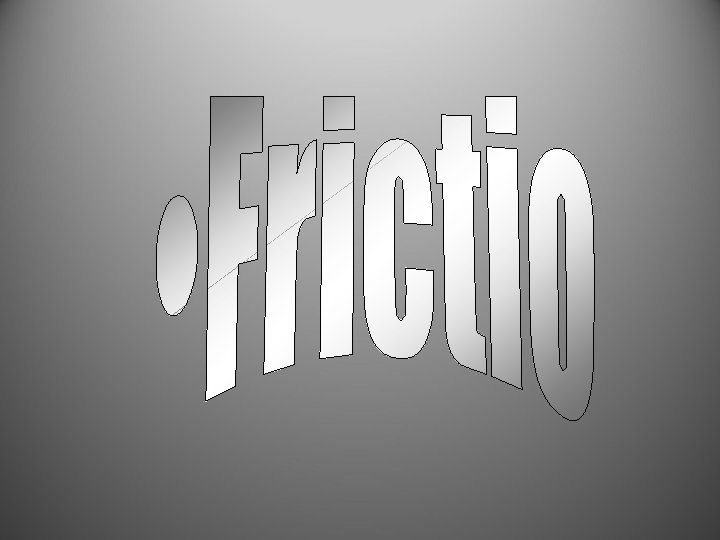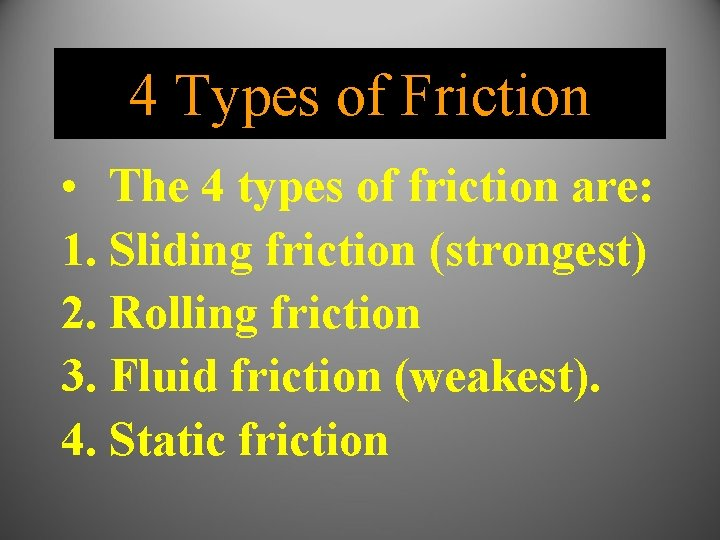4 Types of Friction • The 4 types of friction are: 1. Sliding friction (strongest) 2. Rolling friction 3. Fluid friction (weakest). 4. Static friction• What type of friction?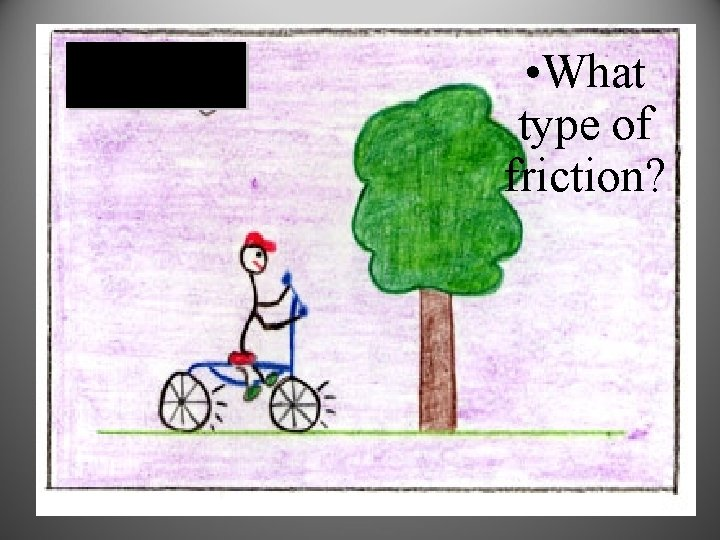• What type of friction?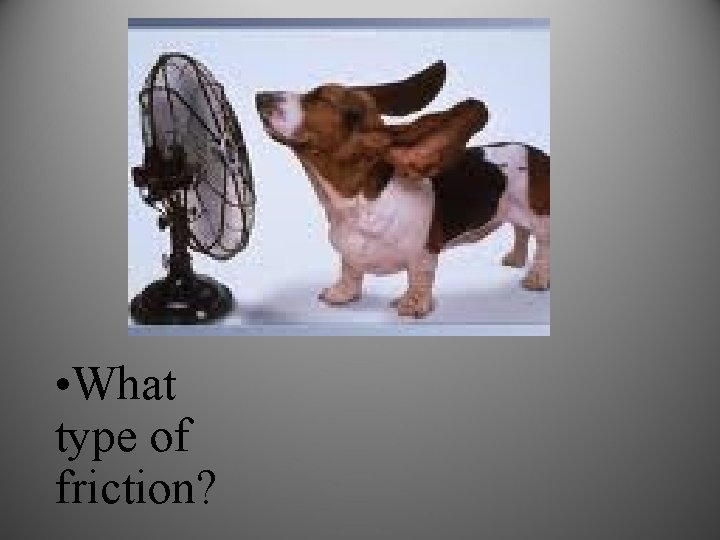• What type of friction?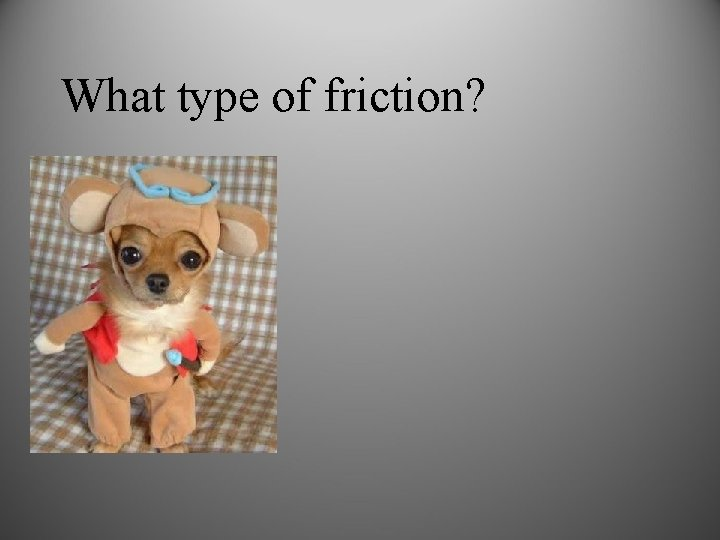What type of friction?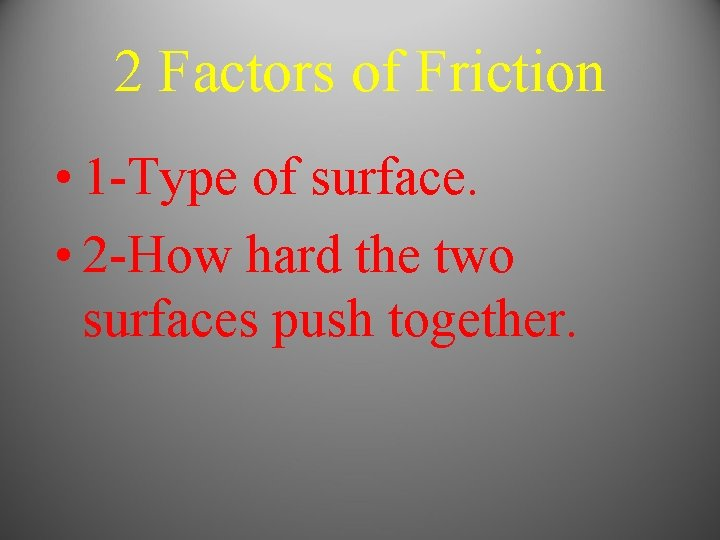2 Factors of Friction • 1 -Type of surface. • 2 -How hard the two surfaces push together.• Which type of hockey has more friction?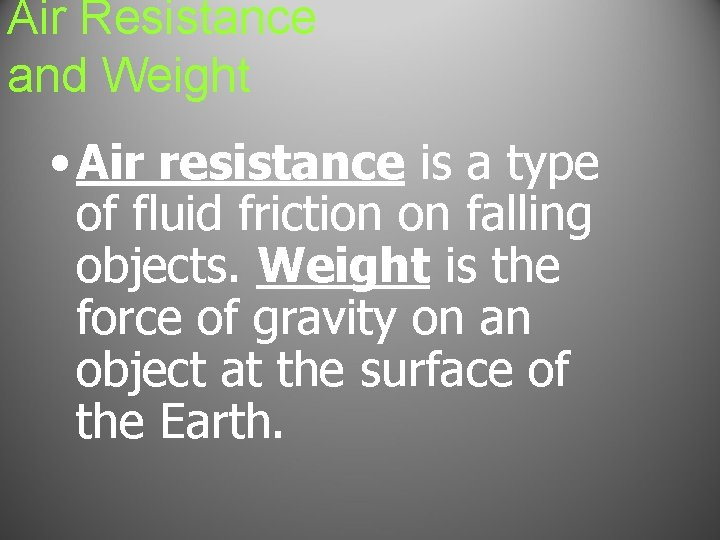Air Resistance and Weight • Air resistance is a type of fluid friction on falling objects. Weight is the force of gravity on an object at the surface of the Earth.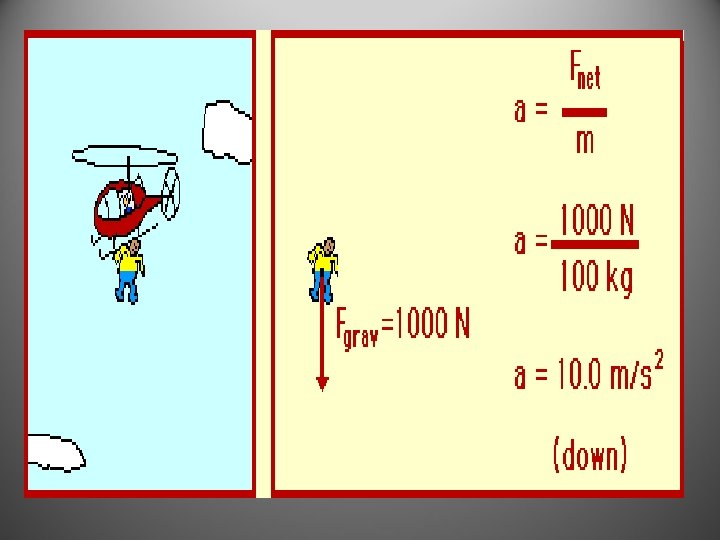Law of Universal Gravitation • Gravity acts between all objects in the universe.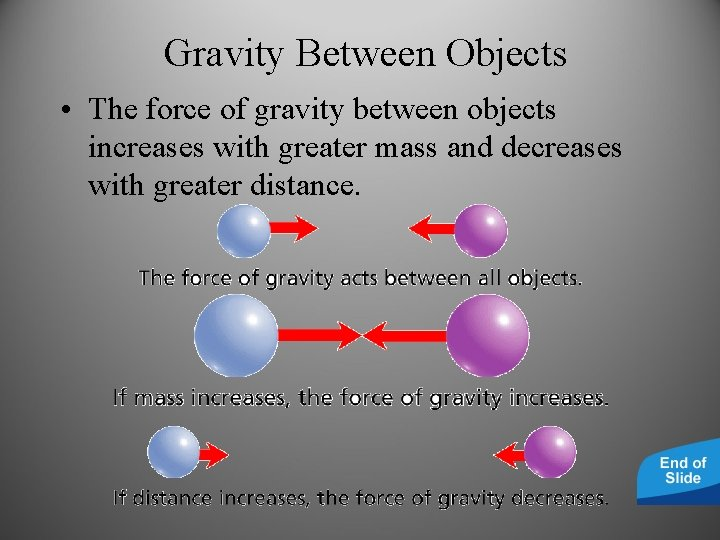Gravity Between Objects • The force of gravity between objects increases with greater mass and decreases with greater distance.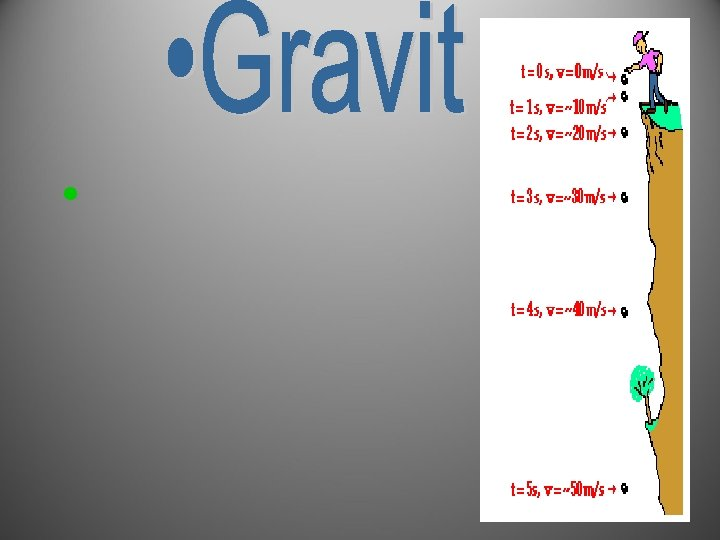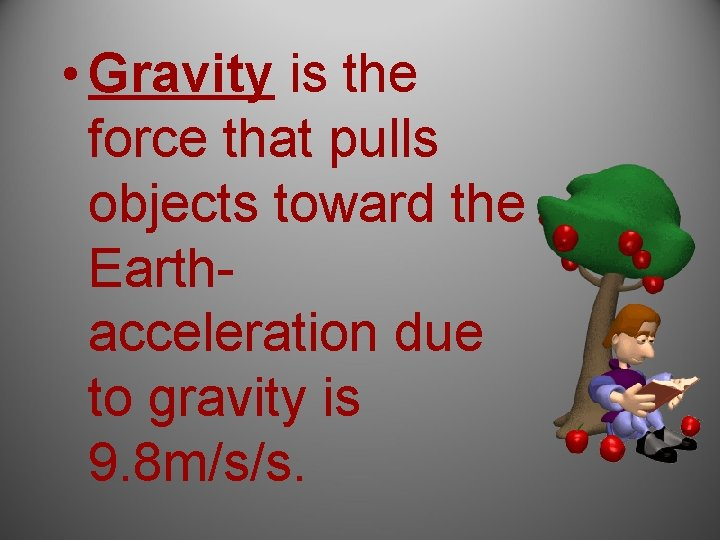• Gravity is the force that pulls objects toward the Earthacceleration due to gravity is 9. 8 m/s/s.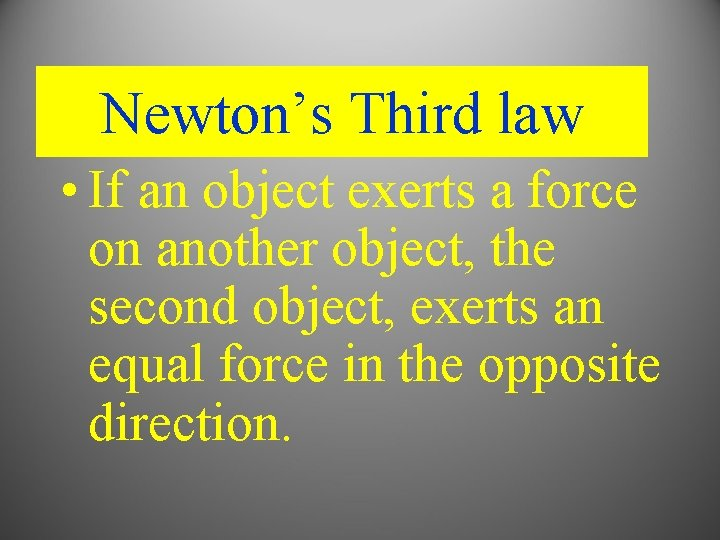Newton’s Third law • If an object exerts a force on another object, the second object, exerts an equal force in the opposite direction.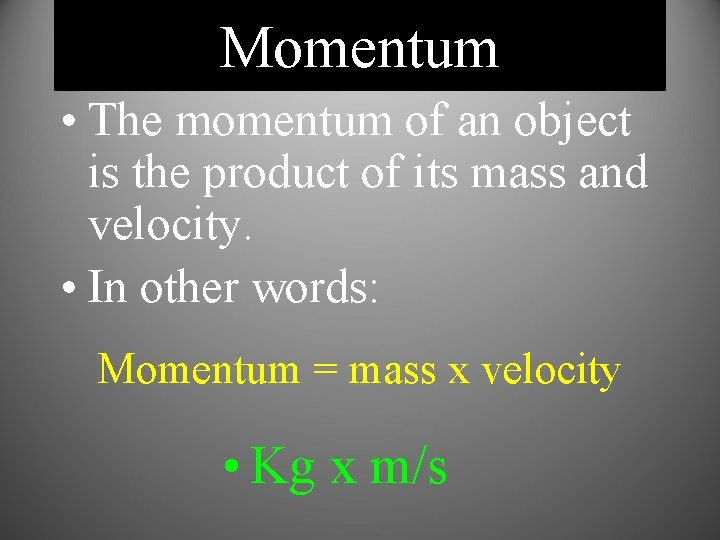Momentum • The momentum of an object is the product of its mass and velocity. • In other words: Momentum = mass x velocity • Kg x m/s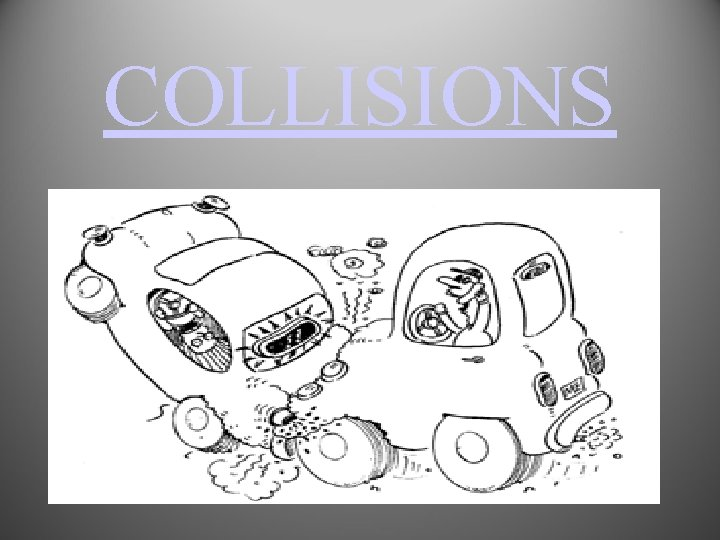COLLISIONS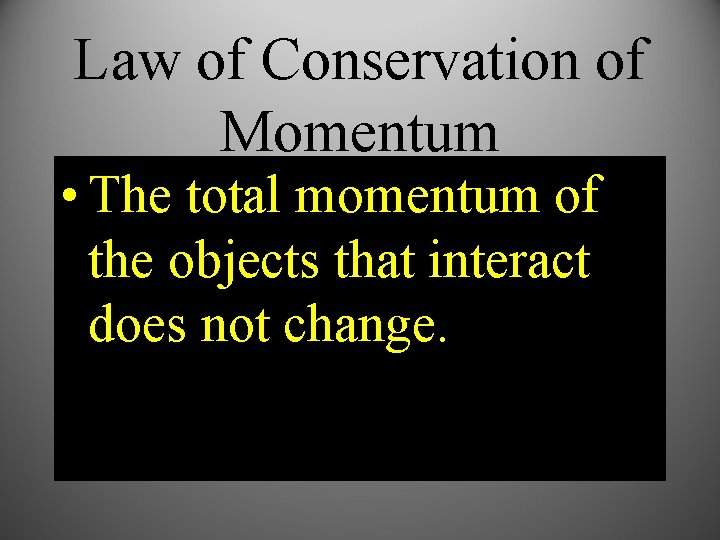Law of Conservation of Momentum • The total momentum of the objects that interact does not change.